PAIREDFREQ Statement

PAIREDFREQ <options> ;

The PAIREDFREQ statement performs power and sample size analyses for McNemar’s test for paired proportions.

### Summary of Options

Table 70.14 summarizes categories of options available in the PAIREDFREQ statement.

Table 70.14 Summary of Options in the PAIREDFREQ Statement

Options

Define analysis

Specify analysis information

Specify effects

Specify sample size

Specify power

Control sample size rounding

Choose computational method

Control ordering in output

Table 70.15 summarizes the valid result parameters in the PAIREDFREQ statement.

Table 70.15 Summary of Result Parameters in the PAIREDFREQ Statement

Analyses

Solve For

Syntax

TEST=MCNEMAR METHOD=CONNOR

Power

Sample size

TEST=MCNEMAR METHOD=EXACT

Power

TEST=MCNEMAR METHOD=MIETTINEN

Power

Sample size

### Dictionary of Options

ALPHA=number-list

specifies the level of significance of the statistical test. The default is 0.05, corresponding to the usual 0.05
100% = 5% level of significance. See the section Specifying Value Lists in Analysis Statements for information about specifying the number-list.

CORR=number-list

specifies the correlation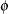between members of a pair. See the section Specifying Value Lists in Analysis Statements for information about specifying the number-list.

DISCPROPORTIONS=grouped-number-list
DISCPS=grouped-number-list

specifies the two discordant proportions,
and
. See the section Specifying Value Lists in Analysis Statements for information about specifying the grouped-number-list.

DISCPROPDIFF=number-list
DISCPDIFF=number-list

specifies the difference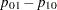between discordant proportions. See the section Specifying Value Lists in Analysis Statements for information about specifying the number-list.

DISCPROPRATIO=number-list
DISCPRATIO=number-list

specifies the ratio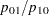of discordant proportions. See the section Specifying Value Lists in Analysis Statements for information about specifying the number-list.

DIST=EXACT_COND
DIST=NORMAL

specifies the underlying distribution assumed for the test statistic. EXACT_COND corresponds to the exact conditional test, based on the exact binomial distribution of the two types of discordant pairs given the total number of discordant pairs. NORMAL corresponds to the conditional test based on the normal approximation to the binomial distribution of the two types of discordant pairs given the total number of discordant pairs. The default value is EXACT_COND.

METHOD=CONNOR
METHOD=EXACT
METHOD=MIETTINEN

specifies the computational method. METHOD=EXACT (the default) uses the exact binomial distributions of the total number of discordant pairs and the two types of discordant pairs. METHOD=CONNOR uses an approximation from Connor (1987), and METHOD=MIETTINEN uses an approximation from Miettinen (1968). The CONNOR and MIETTINEN methods are valid only for DIST=NORMAL.

NFRACTIONAL
NFRAC

enables fractional input and output for sample sizes. See the section Sample Size Adjustment Options for information about the ramifications of the presence (and absence) of the NFRACTIONAL option. This option cannot be used with METHOD=EXACT.

NPAIRS=number-list

specifies the total number of proportion pairs (concordant and discordant) or requests a solution for the number of pairs with a missing value (NPAIRS=.). See the section Specifying Value Lists in Analysis Statements for information about specifying the number-list.

NULLDISCPROPRATIO=number-list
NULLDISCPRATIO=number-list
NULLRATIO=number-list
NULLR=number-list

specifies the null value of the ratio of discordant proportions. The default value is 1. See the section Specifying Value Lists in Analysis Statements for information about specifying the number-list.

ODDSRATIO=number-list
OR=number-list

specifies the odds ratio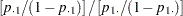. See the section Specifying Value Lists in Analysis Statements for information about specifying the number-list.

OUTPUTORDER=INTERNAL
OUTPUTORDER=REVERSE
OUTPUTORDER=SYNTAX

controls how the input and default analysis parameters are ordered in the output. OUTPUTORDER=INTERNAL (the default) arranges the parameters in the output according to the following order of their corresponding options:

The OUTPUTORDER=SYNTAX option arranges the parameters in the output in the same order in which their corresponding options are specified in the PAIREDFREQ statement. The OUTPUTORDER=REVERSE option arranges the parameters in the output in the reverse of the order in which their corresponding options are specified in the PAIREDFREQ statement.

PAIREDPROPORTIONS=grouped-number-list
PPROPORTIONS=grouped-number-list
PAIREDPS=grouped-number-list
PPS=grouped-number-list

specifies the two paired proportions,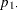and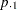. See the section Specifying Value Lists in Analysis Statements for information about specifying the grouped-number-list.

POWER=number-list

specifies the desired power of the test or requests a solution for the power with a missing value (POWER=.). The power is expressed as a probability, a number between 0 and 1, rather than as a percentage. See the section Specifying Value Lists in Analysis Statements for information about specifying the number-list.

PROPORTIONDIFF=number-list
PDIFF=number-list

specifies the proportion difference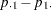. See the section Specifying Value Lists in Analysis Statements for information about specifying the number-list.

REFPROPORTION=number-list
REFP=number-list

specifies either the reference first proportion(when used in conjunction with the PROPORTIONDIFF=, ODDSRATIO=, or RELATIVERISK= option) or the reference discordant proportion(when used in conjunction with the DISCPROPDIFF= or DISCPROPRATIO= option). See the section Specifying Value Lists in Analysis Statements for information about specifying the number-list.

RELATIVERISK=number-list
RR=number-list

specifies the relative risk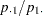. See the section Specifying Value Lists in Analysis Statements for information about specifying the number-list.

SIDES=keyword-list

specifies the number of sides (or tails) and the direction of the statistical test or confidence interval. See the section Specifying Value Lists in Analysis Statements for information about specifying the keyword-list. Valid keywords and their interpretation are as follows:

1

one-sided with alternative hypothesis in same direction as effect

2

two-sided

U

upper one-sided with alternative greater than null value

L

lower one-sided with alternative less than null value

If the effect size is zero, then SIDES=1 is not permitted; instead, specify the direction of the test explicitly in this case with either SIDES=L or SIDES=U. The default value is 2.

TEST=MCNEMAR

specifies the McNemar test of paired proportions. This is the default test option.

TOTALPROPDISC=number-list
TOTALPDISC=number-list
PDISC=number-list

specifies the sum of the two discordant proportions,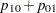. See the section Specifying Value Lists in Analysis Statements for information about specifying the number-list.

### Restrictions on Option Combinations

To specify the proportions, choose one of the following parameterizations:

### Option Groups for Common Analyses

This section summarizes the syntax for the common analyses supported in the PAIREDFREQ statement.

#### McNemar Exact Conditional Test

You can express effects in terms of the individual discordant proportions, as in the following statements. Default values for the TEST=, SIDES=, ALPHA=, and NULLDISCPROPRATIO= options specify a two-sided McNemar test for no effect with a significance level of 0.05.

```proc power;
pairedfreq dist=exact_cond
discproportions = 0.15 | 0.45
npairs = 80
power = .;
run;
```

You can also express effects in terms of the difference and sum of discordant proportions:

```proc power;
pairedfreq dist=exact_cond
discpropdiff = 0.3
totalpropdisc = 0.6
npairs = 80
power = .;
run;
```

You can also express effects in terms of the difference of discordant proportions and the reference discordant proportion:

```proc power;
pairedfreq dist=exact_cond
discpropdiff = 0.3
refproportion = 0.15
npairs = 80
power = .;
run;
```

You can also express effects in terms of the ratio of discordant proportions and the denominator of the ratio:

```proc power;
pairedfreq dist=exact_cond
discpropratio = 3
refproportion = 0.15
npairs = 80
power = .;
run;
```

You can also express effects in terms of the ratio and sum of discordant proportions:

```proc power;
pairedfreq dist=exact_cond
discpropratio = 3
totalpropdisc = 0.6
npairs = 80
power = .;
run;
```

You can also express effects in terms of the paired proportions and correlation:

```proc power;
pairedfreq dist=exact_cond
pairedproportions = 0.6 | 0.8
corr = 0.4
npairs = 45
power = .;
run;
```

You can also express effects in terms of the proportion difference, reference proportion, and correlation:

```proc power;
pairedfreq dist=exact_cond
proportiondiff = 0.2
refproportion = 0.6
corr = 0.4
npairs = 45
power = .;
run;
```

You can also express effects in terms of the odds ratio, reference proportion, and correlation:

```proc power;
pairedfreq dist=exact_cond
oddsratio = 2.66667
refproportion = 0.6
corr = 0.4
npairs = 45
power = .;
run;
```

You can also express effects in terms of the relative risk, reference proportion, and correlation:

```proc power;
pairedfreq dist=exact_cond
relativerisk = 1.33333
refproportion = 0.6
corr = 0.4
npairs = 45
power = .;
run;
```

#### McNemar Normal Approximation Test

The following statements demonstrate a sample size computation for the normal-approximate McNemar test. The default value for the METHOD= option specifies an exact sample size computation. Default values for the TEST=, SIDES=, ALPHA=, and NULLDISCPROPRATIO= options specify a two-sided McNemar test for no effect with a significance level of 0.05.

```proc power;
pairedfreq dist=normal method=connor
discproportions = 0.15 | 0.45
npairs = .
power = .9;
run;
```